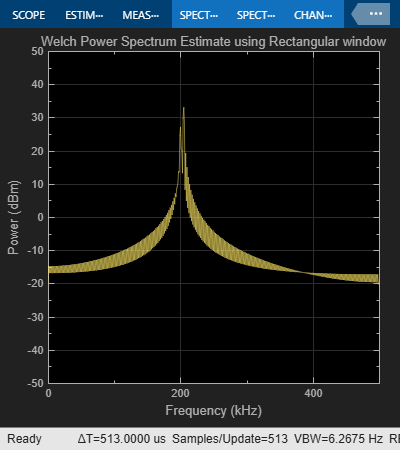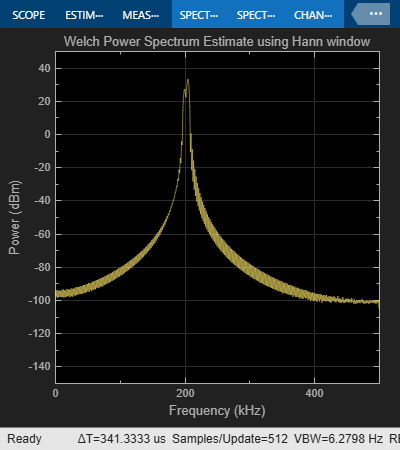# High Resolution Spectral Analysis in Simulink

This example shows how to perform high resolution spectral analysis in Simulink® using the Spectrum Analyzer block and the Spectrum Estimator block.

For comparison purposes, a traditional averaged modified periodogram (Welch's) method is also shown. For a similar example in MATLAB®, see High Resolution Spectral Analysis in MATLAB.

### Using Spectrum Analyzer

The `SpectrumAnalyzerFilterBank` model illustrates the high resolution capabilities of filter bank-based spectral estimation compared to the Welch's method. The filter bank-based spectral estimation has lower noise floor.

Consider the following case. Three sinusoids at 170 kHz, 200 kHz and 205kHz with the amplitudes [1e-5 1 2]. The first sinusoid is completely missed by the rectangular window estimate. The filter bank estimate provides better resolution and better isolation of three tones.

Open and simulate the `SpectrumAnalyzerFilterBank` model.

```open_system('SpectrumAnalyzerFilterBank'); sim('SpectrumAnalyzerFilterBank'); ```Close the model.

```bdclose('SpectrumAnalyzerFilterBank'); ```

### Using Spectrum Estimator

Numerical computations for high resolution spectral estimation shown above can also be modeled in Simulink using the Spectrum Estimator block. The `SpectrumEstimatorFilterBank` model illustrates the high resolution capabilities of filter bank-based spectrum estimation and lower noise floor compared to the Welch's method, using Simulink. Array plot is used to visualize the results. Array plot provides a convenient way of plotting the spectrum estimates. Values are shown in dBm, but Watts or dBW could easily be used instead.

Open and simulate the `SpectrumEstimatorFilterBank` model.

```open_system('SpectrumEstimatorFilterBank'); sim('SpectrumEstimatorFilterBank'); ```Close the model.

```bdclose('SpectrumEstimatorFilterBank'); ```